A reliability evaluation for radionuclide transport analysis code, MATRICS, used in radionuclide transport analysis in the natural barrier system PA in H12 report has been carried out. Sensitivity analysis to radionuclide transport parameter in MATRICS and analytical solution has been performed, and the results of each analysis have been compared. Additionally sensitivity analysis using Talbot Method, Crump method and Hosono method has been carried out, and the results of each inverse Laplace transform method has been compared. The conclusions obtained from the results of the evaluation are summarized as follows, (1)In case of the infinite matrix diffusion distance, an error among the results of each calculation is maximum about 0.4% in the range of Pe number from 1.0 to 100. And, an error among the results of each calculation is maximum about 5.5% in the range of transmissivity from 1.0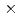10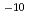to 1.010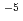(m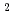/s). (2)In case of the finite matrix diffusion distance (0.03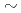1.0(m)), an error among the results of each calculation is maximum about 0.7% in the range of Pe number from 1.0 to 100. And, an error among the results of each calculation is maximum about 2.4% in the range of transmissivity from 1.010to 1.010(m/s). 3)By comparing Talbot method with other inverse Laplace transform method, Talbot method is confirmed to give similar results with other inverse Laplace transform method in the range of Pe number from 5.010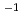to 2.010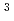, and that of transmissivity below 1.010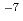(m/s). Therefore, it is concluded that the reliability of MATRICS are confirmed by conducting sensitivity analysis in the range of Pe number and transmissivity coefficient used in H12 report.